# sknano.generators.SWNTBundleGenerator¶

class sknano.generators.SWNTBundleGenerator(autogen=True, **kwargs)[source][source]

Class for generating nanotube bundles.

New in version 0.2.4.

Parameters: *Ch : {tuple or ints} Either a 2-tuple of ints or 2 integers giving the chiral indices of the nanotube chiral vector $$\mathbf{C}_h = n\mathbf{a}_1 + m\mathbf{a}_2 = (n, m)$$. nx, ny, nz : int, optional Number of repeat unit cells in the $$x, y, z$$ dimensions. basis : {list}, optional List of strs of element symbols or atomic number of the two atom basis (default: [‘C’, ‘C’]) New in version 0.3.10. element1, element2 : {str, int}, optional Element symbol or atomic number of basis Atom 1 and 2 Deprecated since version 0.3.10: Use basis instead bond : float, optional $$\mathrm{a}_{\mathrm{CC}} =$$ distance between nearest neighbor atoms. Must be in units of Angstroms. vdw_radius : float, optional van der Waals radius of nanotube atoms New in version 0.2.5. bundle_packing : {‘hcp’, ‘ccp’}, optional Packing arrangement of nanotubes bundles. If bundle_packing is None, then it will be determined by the bundle_geometry parameter if bundle_geometry is not None. If both bundle_packing and bundle_geometry are None, then bundle_packing defaults to hcp. New in version 0.2.5. bundle_geometry : {‘triangle’, ‘hexagon’, ‘square’, ‘rectangle’}, optional Force a specific geometry on the nanotube bundle boundaries. New in version 0.2.5. Lz : float, optional length of bundle in $$z$$ dimension in nanometers. Overrides nz value. New in version 0.2.5. fix_Lz : bool, optional Generate the nanotube with length as close to the specified $$L_z$$ as possible. If True, then non integer $$n_z$$ cells are permitted. New in version 0.2.6. autogen : bool, optional if True, automatically generate structure data. verbose : bool, optional if True, show verbose output

Examples

Using the SWNTBundleGenerator class, you can generate structure data for nanotube bundles with either cubic close packed (ccp) or hexagonal close packed (hcp) arrangement of nanotubes. The bundle packing arrangement is set using the bundle_packing parameter.

You can also enforce a specific bundle geometry which will try and build the nanotube bundle such that it “fits inside” the boundaries of a specified geometric shape. This allows you to generate hcp bundles that are trianglar, hexagonal, or rectangular in shape, as some of the examples below illustrate.

To start, let’s generate an hcp bundle of $$C_{\mathrm{h}} = (10, 5)$$ SWCNTs and cell count $$n_x=10, n_y=3, n_z=5$$.

>>> from sknano.generators import SWNTBundleGenerator
>>> SWCNTbundle = SWNTBundleGenerator(n=10, m=5, nx=10, ny=3, nz=5)
>>> SWCNTbundle.save()


The rendered structure looks like: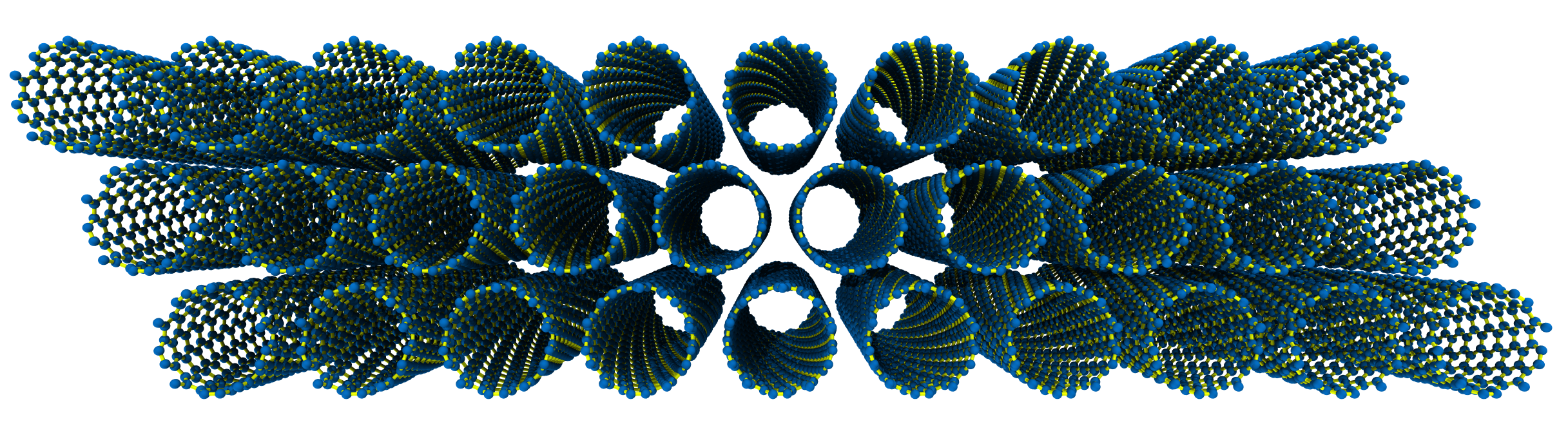Now let’s generate a nice hexagon bundle, 3 tubes wide, with $$C_{\mathrm{h}} = (6, 5)$$.

>>> SWCNTbundle = SWNTBundleGenerator(n=6, m=5, nz=5,
...                                   bundle_geometry='hexagon')
>>> SWCNTbundle.save()


which looks like: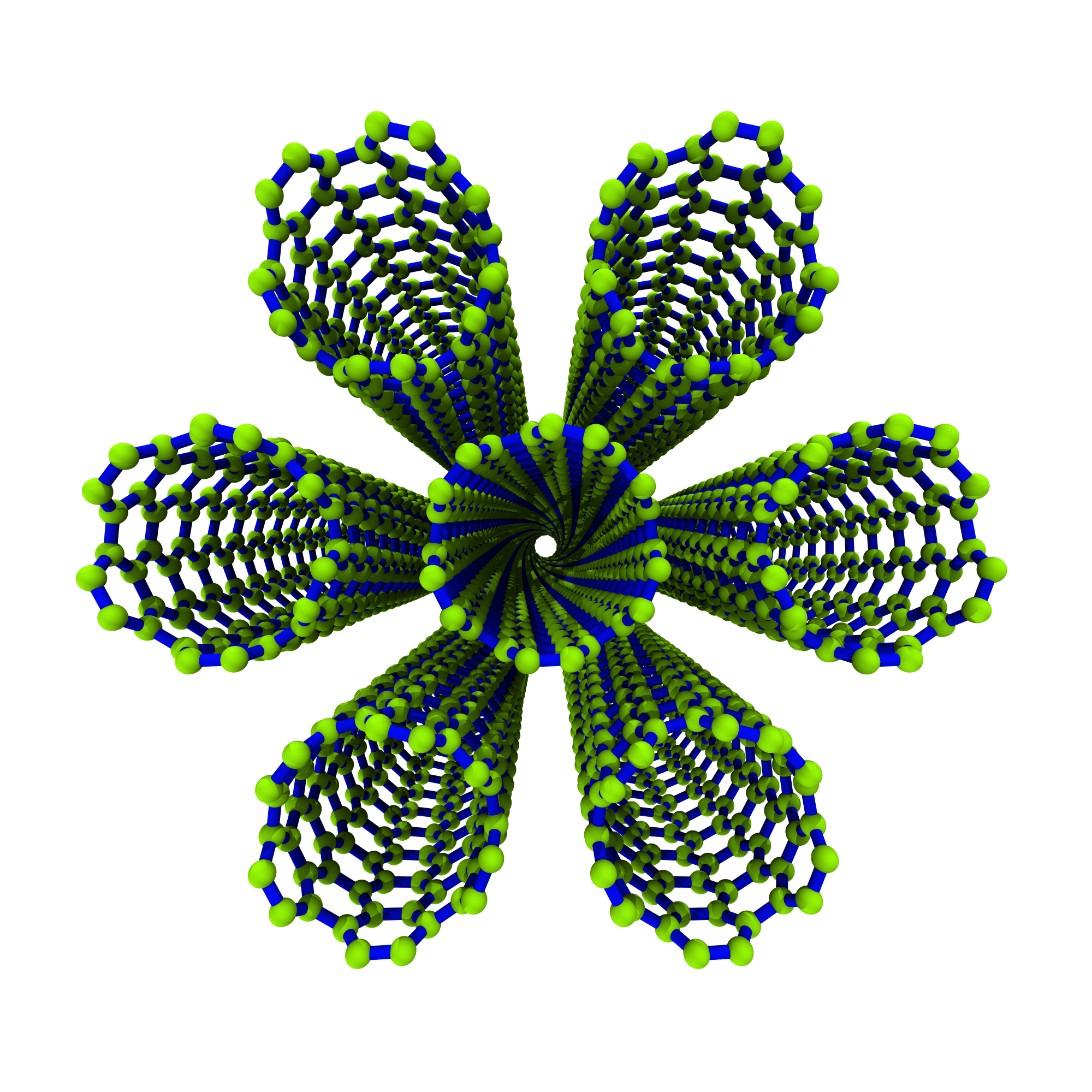Remember, all of the save methods allow you to rotate the structure data before saving:

>>> SWCNTbundle.save(fname='0605_hcp_7tube_hexagon_rot-30deg.xyz',
...                  rot_axis='z', rotation_angle=30)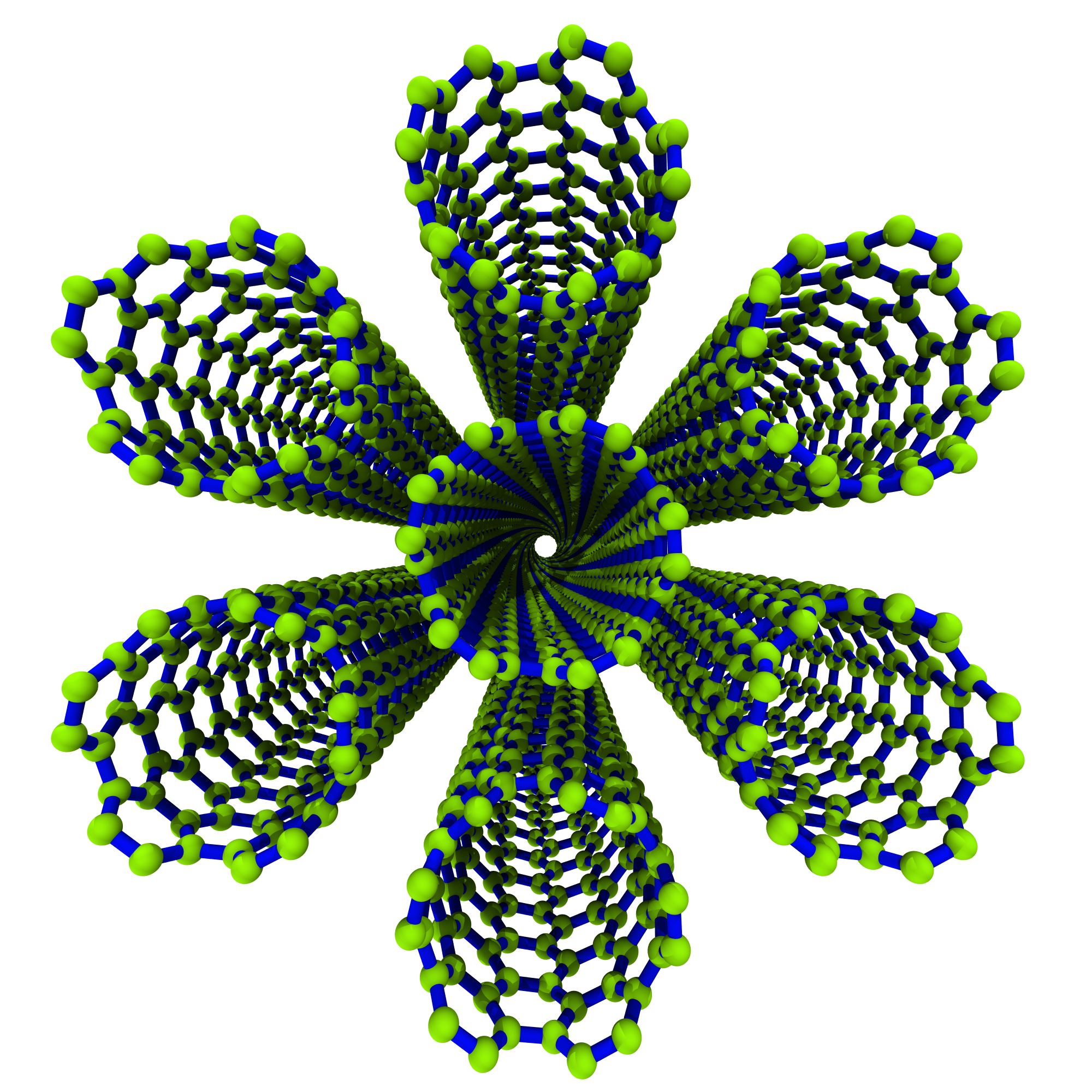Now, just because we can, let’s make a big ass hexagon bundle with $$C_{\mathrm{h}} = (10, 0)$$.

>>> big_hexagonal_bundle = SWNTBundleGenerator(10, 0, nx=25, ny=25, nz=1,
...                                            bundle_geometry='hexagon')
>>> big_hexagonal_bundle.save()


Take a look at the 469 $$(10, 0)$$ unit cells in this big ass bundle!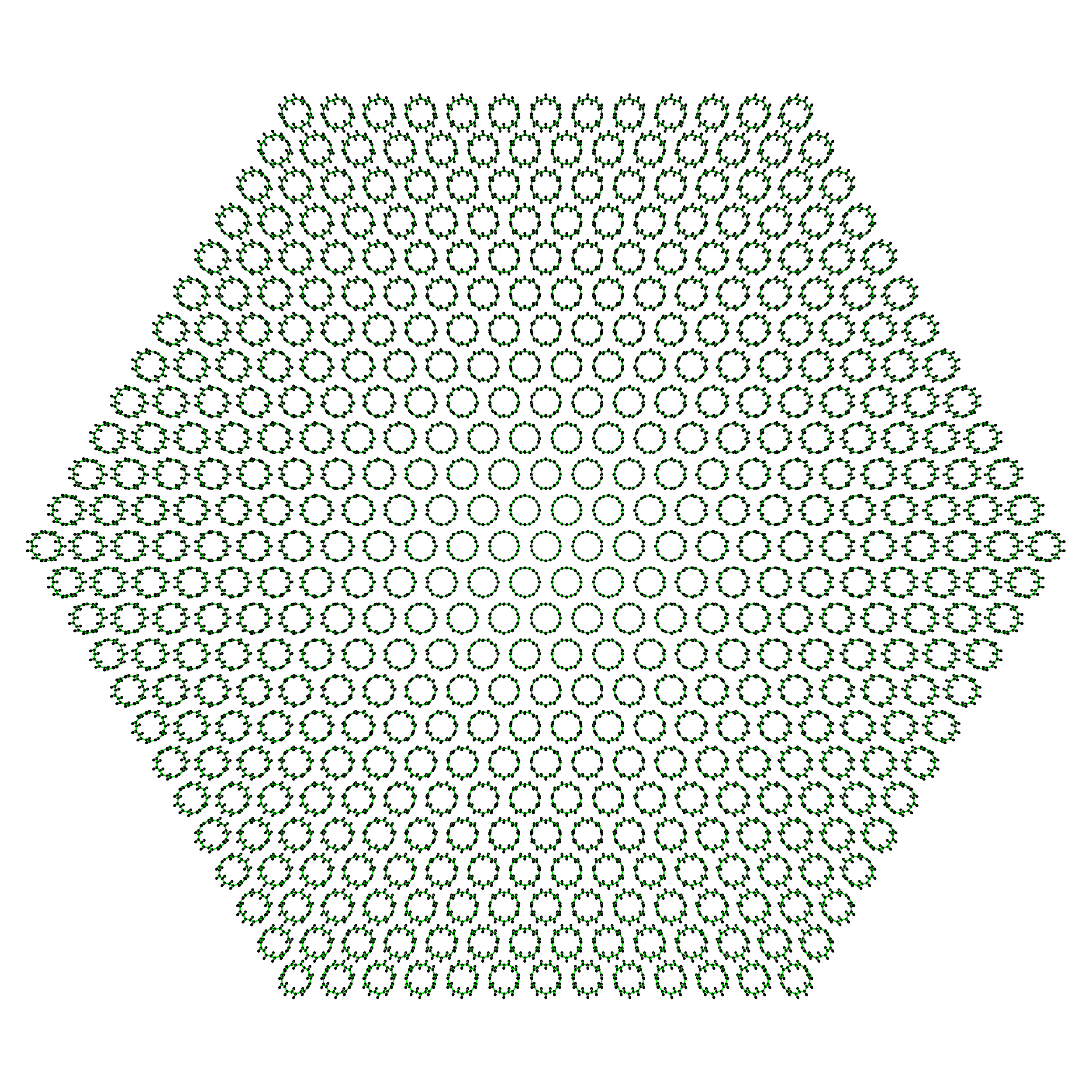Lastly, here’s a look at a bundle generated with cubic close packed bundle arrangement:

>>> SWCNTbundle = SWNTBundleGenerator(10, 10, nx=3, ny=3, nz=5,
...                                   bundle_packing='ccp')
>>> SWCNTbundle.save()


The rendered ccp structure looks like: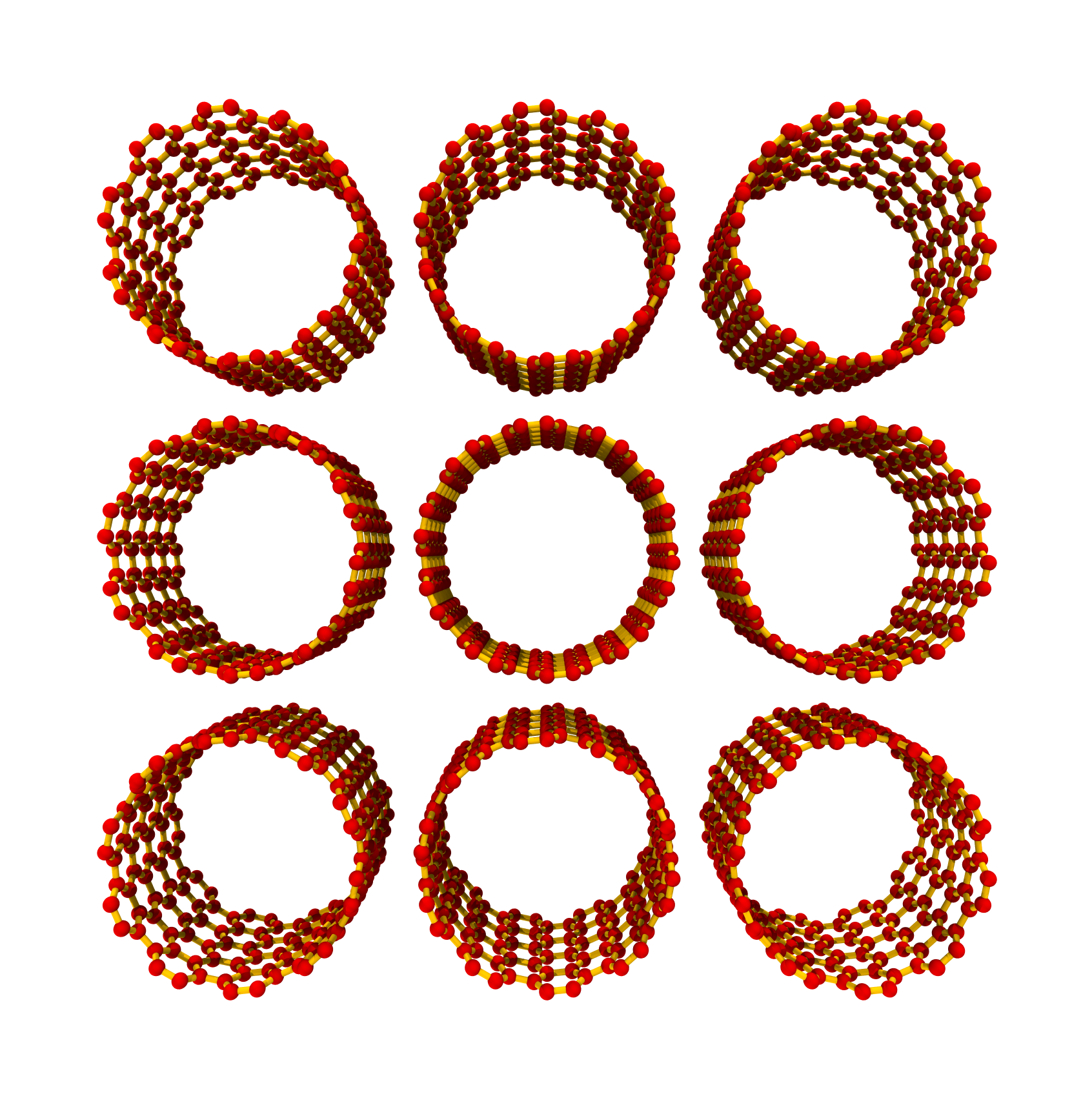Attributes

 Ch SWNT circumference $$|\mathbf{C}_h|$$ in Å Ch_vec SWNT chiral vector. Lx Ly Lz SWNT length $$L_z = L_{\mathrm{tube}}$$ in nanometers. M $$M = np - nq$$ N Number of graphene hexagons in nanotube unit cell. Natoms Number of atoms in nanotube bundle. Natoms_list Natoms_per_bundle Natoms_per_tube Alias for Natoms_list. Natoms_per_unit_cell Number of atoms in nanotube unit cell. Ntubes R Symmetry vector $$\mathbf{R} = (p, q)$$. T Length of nanotube unit cell $$|\mathbf{T}|$$ in Å. Tvec SWNT translation vector. atoms Structure StructureAtoms. basis NanoStructureBase basis atoms. bundle_density bundle_geometry bundle_mass bundle_packing chiral_angle Chiral angle $$\theta_c$$ in degrees. chiral_type SWNT chiral type. crystal_cell Structure CrystalCell. d $$d=\gcd{(n, m)}$$ dR $$d_R=\gcd{(2n + m, 2m + n)}$$ dt Nanotube diameter $$d_t = \frac{|\mathbf{C}_h|}{\pi}$$ in Å. electronic_type SWNT electronic type. element1 Basis element 1 element2 Basis element 2 fix_Lz fmtstr Format string. lattice Structure Crystal3DLattice. linear_mass_density Linear mass density of nanotube in g/nm. m Chiral index $$m$$. n Chiral index $$n$$. nx Number of nanotubes along the $$x$$-axis. ny Number of nanotubes along the $$y$$-axis. nz Number of nanotube unit cells along the $$z$$-axis. rt Nanotube radius $$r_t = \frac{|\mathbf{C}_h|}{2\pi}$$ in Å. scaling_matrix CrystalCell.scaling_matrix. structure Pointer to self. structure_data Alias for BaseStructureMixin.structure. t1 $$t_{1} = \frac{2m + n}{d_{R}}$$ t2 $$t_2 = -\frac{2n + m}{d_R}$$ tube_length Alias for SWNT.Lz tube_mass SWNT mass in grams. unit_cell Structure UnitCell. unit_cell_mass Unit cell mass in atomic mass units. unit_cell_symmetry_params Tuple of SWNT unit cell symmetry parameters. vdw_distance van der Waals distance. vdw_radius van der Waals radius

Methods

 clear() Clear list of BaseStructureMixin.atoms. generate([generate_bundle, ...]) Generate structure data. generate_bundle() generate_bundle_coords() Generate coordinates of bundle tubes. generate_bundle_from_bundle_coords() generate_fname([n, m, nx, ny, nz, fix_Lz, ...]) generate_unit_cell() Generate the nanotube unit cell. make_supercell(scaling_matrix[, wrap_coords]) Make supercell. read_data(*args, **kwargs) read_dump(*args, **kwargs) read_xyz(*args, **kwargs) rotate(**kwargs) Rotate crystal cell lattice, basis, and unit cell. save([fname, outpath, structure_format, ...]) Save structure data. todict() transform_lattice(scaling_matrix[, ...]) translate(t[, fix_anchor_points]) Translate crystal cell basis. write_data(**kwargs) write_dump(**kwargs) write_xyz(**kwargs)